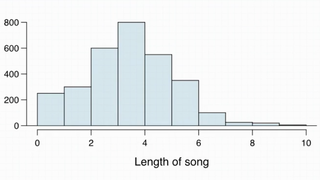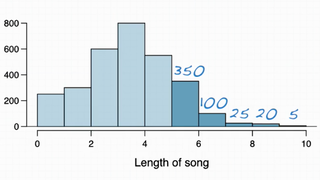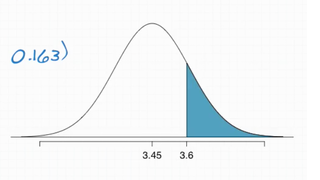### Example 1

Suppose my iPod has 3,000 songs. The histogram below shows the distribution of the lengths of these songs. We also know that, for this iPod, the mean length is 3.45 minutes and the standard deviation is 1.63 minutes. Calculate the probability that a randomly selected song lasts more than 5 minutes.This is the same thing as saying among all the population of songs on this iPod, what percentage of them last more than 5 minutes. It seems we can calculate Z-score and the associated normal probabilities to find probability. However, this distribution is not nearly normal, it is indeed right-skewed (Because there is no upper end to how long your songs can be).

Note: To use Z-scores and the associated normal probabilities, you need to make sure the distribution we work with is nearly normal.

To do this, we need to make some educated guess:Therefore,

```                      350 + 100 + 25 + 20 + 5    500
P(song length > 5) = ------------------------ = ----- = 0.17
3000                  3000```

So, 17% of songs in my iPod last more than 5 minutes

### Example 2

I am about to take a trip to visit my parentsand the drive is 6 hours. I make a random playlist of 100 songs. What is the probability that my playlist lasts the enire drive?

We know 6 hours is 360 minutes, so we need 360 minutes worth of songs. Therefore, we can write this question to

`P( X1 + X2 + X3 + ... + X100 > 360 min) = ? `

This is equivalent to the average length > 3.6 minutes:

`P( x̄ > 3.6 ) = ? `

The central limit theorem says that x̄ will be distributed nearly normally when mean = population mean, which is 3.45 minutes. And with standard error equal to the population standard deviation σ divided by √n

```                              σ    1.63
x̄ ~ N (mean = μ = 3.45, SE = --- = ----- = 0.163)
√n    √100```

So, we draw the curve and we are looking for everything above that:Next, we calculate the Z score:

```      3.6 - 3.45
Z = ------------- = 0.92
0.163```

After that, we can easily find the probability using central limit theorem, R or web app.

`P( X > 0.92 ) = 0.179`

So there is about 18% chance that my playlist lasts at least the entire drive.

• N/A